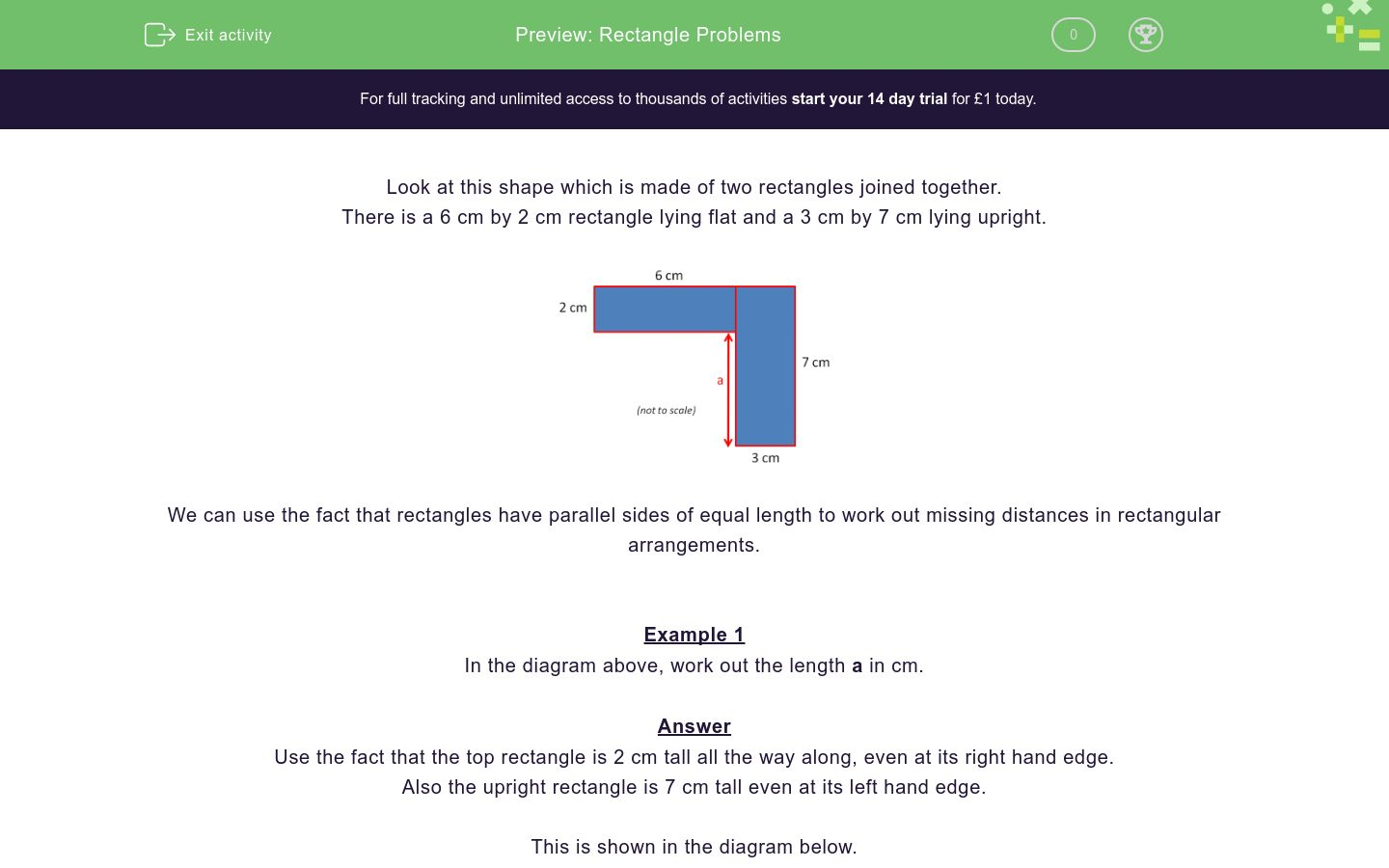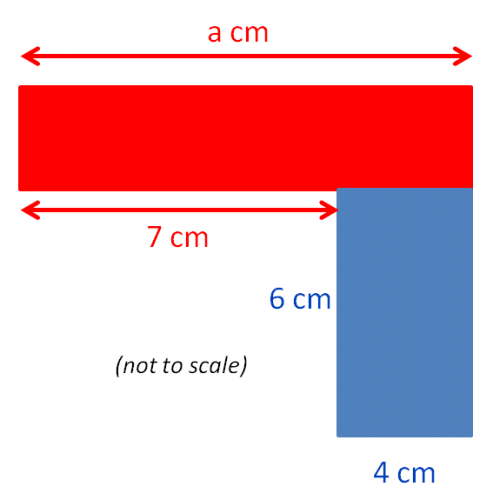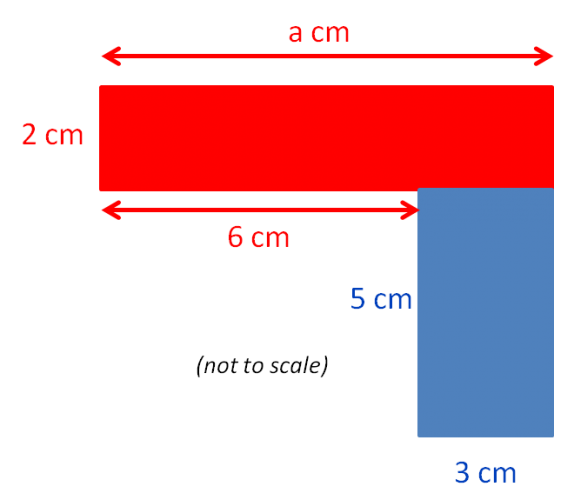# Rectangle Problems

In this worksheet, students use the properties of rectangles to find missing lengths in rectangular shapes.Key stage:  KS 2

Curriculum topic:   Geometry: Properties of Shapes

Curriculum subtopic:   Understand Rectangles

Difficulty level:### QUESTION 1 of 10

Look at this shape which is made of two rectangles joined together.

There is a 6 cm by 2 cm rectangle lying flat and a 3 cm by 7 cm lying upright.We can use the fact that rectangles have parallel sides of equal length to work out missing distances in rectangular arrangements.

Example 1

In the diagram above, work out the length a in cm.

Use the fact that the top rectangle is 2 cm tall all the way along, even at its right hand edge.

Also the upright rectangle is 7 cm tall even at its left hand edge.

This is shown in the diagram below.This means that a is 7 cm - 2 cm = 5 cm.

Example 2

In the diagram, work out the length a in cm.Use the fact that the top of the blue rectangle will match the length of the bottom as 4 cm.

The length a will be 7 cm + 4 cm.

This is shown in the diagram below.This means that a is 7 cm + 4 cm = 11 cm.

The diagram shows two rectangles.Work out the length a in cm.

(Just write the number.)

The diagram shows two rectangles.Work out the length a in cm.

(Just write the number.)

The diagram shows two rectangles.Work out the length a in cm.

(Just write the number.)

The diagram shows two rectangles.Work out the length a in cm.

(Just write the number.)

The diagram shows two rectangles.Work out the length a in cm.

(Just write the number.)

The diagram shows two rectangles.Work out the length a in cm.

(Just write the number.)

The diagram shows two rectangles.Work out the length a in cm.

(Just write the number.)

The diagram shows two rectangles.Work out the length a in cm.

(Just write the number.)

The diagram shows two rectangles.Work out the length a in cm.

(Just write the number.)

The diagram shows two rectangles.Work out the length a in cm.

(Just write the number.)

• Question 1

The diagram shows two rectangles.Work out the length a in cm.

(Just write the number.)

6
EDDIE SAYS
9 - 3 = 6 cm
• Question 2

The diagram shows two rectangles.Work out the length a in cm.

(Just write the number.)

9
EDDIE SAYS
6 + 3 = 9 cm
• Question 3

The diagram shows two rectangles.Work out the length a in cm.

(Just write the number.)

13
EDDIE SAYS
18 - 5 = 13 cm
• Question 4

The diagram shows two rectangles.Work out the length a in cm.

(Just write the number.)

16
EDDIE SAYS
11 + 5 = 16 cm
• Question 5

The diagram shows two rectangles.Work out the length a in cm.

(Just write the number.)

9
EDDIE SAYS
14 - 5 = 9 cm
• Question 6

The diagram shows two rectangles.Work out the length a in cm.

(Just write the number.)

5
EDDIE SAYS
8 - 3 = 5 cm
• Question 7

The diagram shows two rectangles.Work out the length a in cm.

(Just write the number.)

6
EDDIE SAYS
20 - 14 = 6 cm
• Question 8

The diagram shows two rectangles.Work out the length a in cm.

(Just write the number.)

11
EDDIE SAYS
25 - 14 = 11 cm
• Question 9

The diagram shows two rectangles.Work out the length a in cm.

(Just write the number.)

5
EDDIE SAYS
12 - 7 = 5 cm
• Question 10

The diagram shows two rectangles.Work out the length a in cm.

(Just write the number.)

23
EDDIE SAYS
18 + 5 = 23 cm
---- OR ----

Sign up for a £1 trial so you can track and measure your child's progress on this activity.

### What is EdPlace?

We're your National Curriculum aligned online education content provider helping each child succeed in English, maths and science from year 1 to GCSE. With an EdPlace account you’ll be able to track and measure progress, helping each child achieve their best. We build confidence and attainment by personalising each child’s learning at a level that suits them.

Get started